Smartick is a fun way to learn math!Nov17

# Geometry and 2D Shapes with the Help of a Tangram

In today’s post we’re going to talk about Geometry and and 2D figures.  To help us, we’re going to use an old Chinese game, Tangram.

The Tangram is a puzzle made up of 7 pieces: a parallelogram (rhomboid), a square, and 5 triangles. The objective of this game is to create shapes using the 7 pieces. The pieces must touch but not overlap.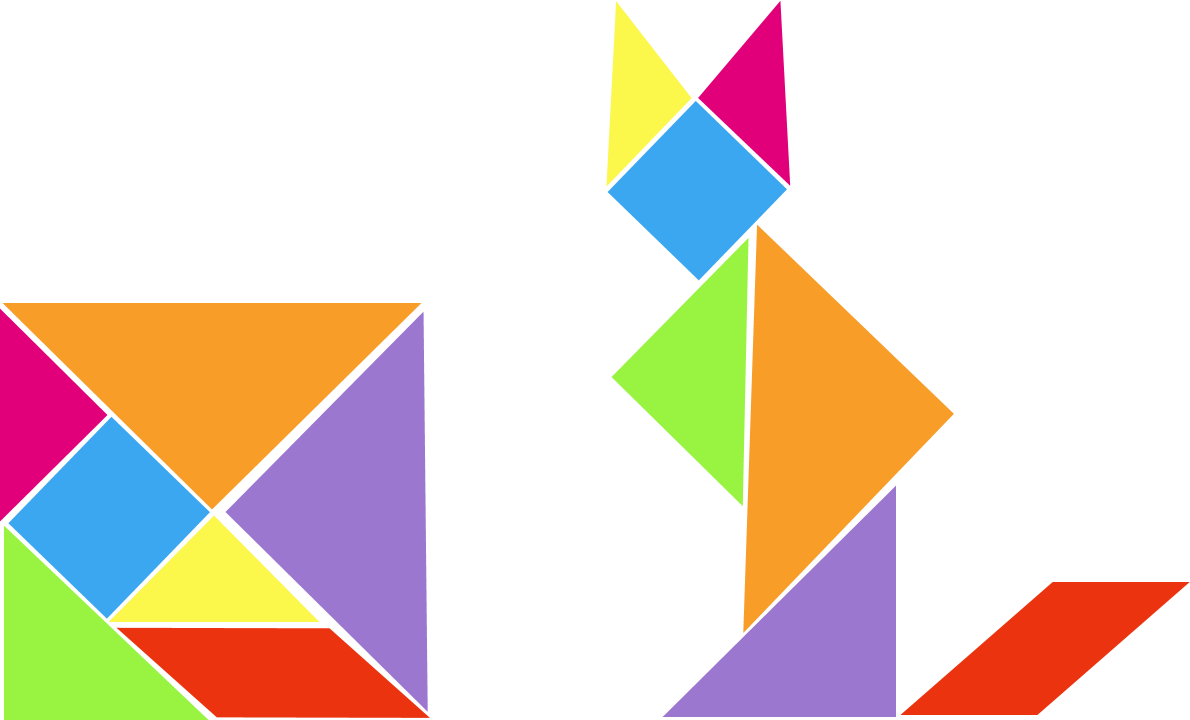Working with the Tangram, apart from stimulating creativity and developing spatial vision, deepens knowledge of different mathematical areas, geometry in particular. It can be used to introduce concepts of 2D geometry.

### Let’s take a look at the shapes we use with the Tangram:

Triangle: A polygon with three sides and three angles.Square: A polygon with four equal sides and four right angles.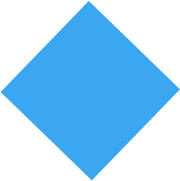Parallelogram: A polygon with four sides, which has two sets of equal sides and two sets of equal angles.At Smartick we go one step further and introduce more shapes, so we can make almost everything we can imagine. Let’s take a look at what 2D geometrical figures the Smartick Rooster is made up of: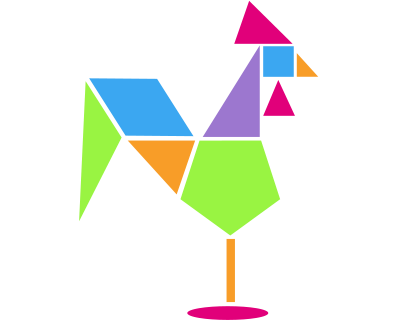The Smartick Rooster contains 1 square: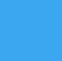If you count, you will find that the Smartick Rooster is made up of 6 triangles: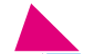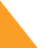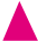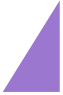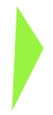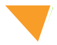There’s also 1 rhomboid:The Smartick Rooster’s belly is made from a pentagon. A pentagon is a 2D geometric figure with five sides and five equal angles.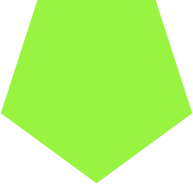Its legs are made from a rectangle. A rectangle is a parallelogram that has two sets of equal sides and four right angles.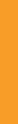The feet are made from an oval. An oval is a squashed circle that represents an ovoid or elliptical shape.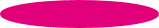You could make your own Tangram with all these 2D figures!!

There are many more 2D shapes you can find if you investigate… Take a look at our previous posts to see if you can find any more shapes than the ones we’ve seen today:

If you want to keep learning primary mathematics, register with Smartick and try it for free.

Fun is our brain’s favorite way of learning
Diane Ackerman
Smartick is a fun way to learn math
• 15 fun minutes a day